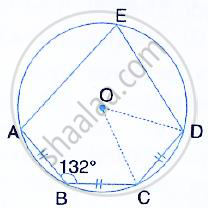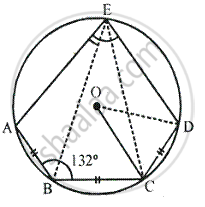Share

# In the Given Figure, Ab = Bc = Cd and ∠Abc = 132 . Calcualte: - Mathematics

Course

#### Question

In the given figure, AB = BC = CD and ∠ABC = 132° . Calcualte: ∠AEB#### SolutionIn the figure, O is the centre of circle, with AB = BC = CD.
Also, ∠ABC = 132°

∠ABC + ∠AEC = 180°               [sum of opposite angles]
→ ∠132 + ∠AEC = 180°
→ ∠AEC = 180° -132°
→ ∠AEC = 48°

Since, AB = BC, ∠AEB = ∠BEC          [equal chords subtends equal angles]

∴ ∠AEB = 1 /2 ∠AEC
= 1 /2xx 48°
= 24°

Is there an error in this question or solution?